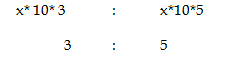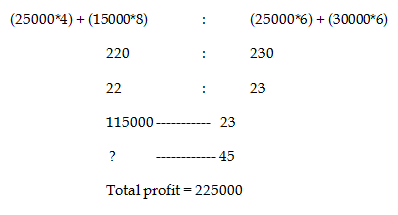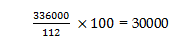# RRB ALP 2018 Practice Test Papers | Arithmetic Questions (Day-84)

Dear Aspirants, Here we have given the Important RRB ALP & Technicians Exam 2018 Practice Test Papers. Candidates those who are preparing for RRB ALP 2018 can practice these Arithmetic Questions to get more confidence to Crack RRB 2018 Examination.

[WpProQuiz 3195]

Click “Start Quiz” to attend these Questions and view Solutions

1) A number is 36 more than its three-seventh. What is the number?

a) 6

b) 7

c) 3

d) 9

2) Find the value of

9522 ÷ 6 ÷ 3 = ?

a) 530

b) 546

c) 529

d) 456

3) 3/ 4 of 2/ 5 of 7/ 8 of x = 21. Find the value of x

a) 20

b) 40

c) 80

d) 79

4)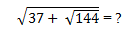a) 7

b) 8

c) 9

d) 4

5) The Average of 3 consecutive numbers is 50. Find the smallest number

a) 50

b) 48

c) 49

d) 51

6) A’s is income in 20 % less than B’s income. C is income in 25% more than B’s Income. What is the ratio of income of A:C?

a) 5 : 4

b) 16 : 25

c) 36 :25

d) 34 : 25

7) Raman has some mangoes and Apples. He had 25% of apple as same as 1/3rd of Mango. Find the ratio of Apple is that of Mango?

a) 2 : 3

b) 2 : 5

c) 4: 3

d) 4 : 5

8) What is the ratio of Simple Interest earned on certain amount at the rate of 10 % per year for 3 years and 5 years?

a) 2: 5

b) 5 : 3

c) 3 : 5

d) 3 : 4

9) Two friends A and B started a business with the investment amount of 25000 each after 4 months A withdrew his 2/5th amount and after 6 months B added 5000 in the business investment. At the end of one year B earns 115000 as his profit. Find the total profit earned by them.

a) 125000

b) 225000

c) 125340

d) 24550

10) Kiran sold a television for Rs. 336000 at the gain of 12%. Find the cost price of the Television.

a) 45000

b) 40000

c) 34000

d) 30000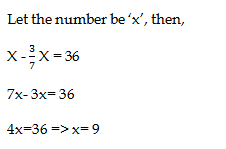9522 × 1/ 6 × 1/ 3 = 529

X = 21 * (4/3) * (5/2) * (8/7) = 80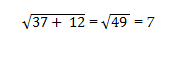X -1 + x + x + 1 = 50* 3

3x = 150

X = 50

x-1 =  49

Smallest number = 49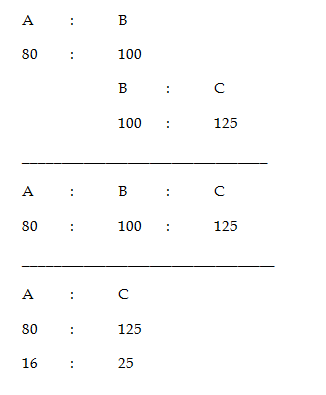25% of apple = 1/3 Mango

25/100 Apple = 1/3 Mango

1/4 Apple = 1/3 Mango

3 Apple = 4 Mango

Apple : Mango = 4 : 3

Ratio of simple interest